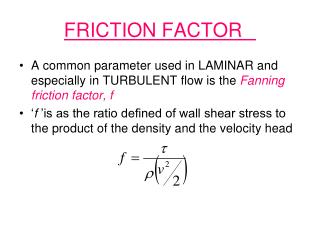DownloadDownload PresentationFRICTION FACTOR

# FRICTION FACTOR

Télécharger la présentation## FRICTION FACTOR

- - - - - - - - - - - - - - - - - - - - - - - - - - - E N D - - - - - - - - - - - - - - - - - - - - - - - - - - -
##### Presentation Transcript

1. FRICTION FACTOR • A common parameter used in LAMINAR and especially in TURBULENT flow is the Fanning friction factor, f • ‘f ’is as the ratio defined of wall shear stress to the product of the density and the velocity head

2. General Eqn for “f” We know that, for flow of fluid in a circular tube, Sub. the above eqn in the previous one… For laminar flow, by comparing above two equations,…. For LAMINAR flow

3. F.L in B.Eqn • In a straight pipe…..the friction losses to be used in B.Eqn is….. • We know, • But all the terms in B.Eqn are having the units of J/kg…..so friction losses is written by modifying the above eqn as…

4. FRICTION FACTOR---TURBULENT FLOW • In turbulent flow, it is not possible to predict the value of ‘f ’ theoretically • It should be determined empirically (experimentally) • It also depends on surface roughness of the pipe. • To predict the value ‘f ’ …. Friction factor chart (MOODY chart) is available ‘f’ vs NRe

5. Friction factor chart (MOODY chart)

6. Relative roughness factors ε/D where ‘ε’ is the roughness parameter, represents the average ht in ‘m’ for projections from the wall • For commercial steel pipe, ε = 4.6 x 10-5m

7. Prob 1 • Water flows in a smooth plastic pipe of 200mm dia @ a rate of 0.1m3/s. Det the friction factor for this flow: • Re = 6.36x105------Turbulent flow • For smooth pipe, ε/D = 0 • From Moody’s chart….. • f = 0.003

8. Prob 2 • Calculate the press. drop along 170m of 5cm dia, horizontal steel pipe thro which olive oil at 20ºC is flowing at a rate of 0.1m3/min. density 910kg/m3 & viscosity 84x10-3 Ns/m2 • We know, • NRe = 460 • For Laminar flow, f = (16/NRe )= 0.0347 • ∆P=154.709kPa

9. Prob 3 • Water is to flow thro 300m of horizontal pipe at a rate of 0.06 m3/s. A head of 6m is available. What must be the pipe dia? Take f = 0.0056 • We know, • v =(Q/A)= 0.0764/D2 • and ∆P = hrg • D = 0.201cm